Categories

# Sizeof operator in C language

The Sizeof is an important unary operator in the C programming language. It helps us in determining the size of primitive data types, user-defined data types, expressions, etc. in computer memory. The Sizeof operator plays an important role in allocating dynamic memory in C using malloc, calloc, etc. in the Heap memory. How Sizeof operator works in C language is explained in this article.

In this article, we are going to learn about the sizeof operator in C. It is a widely used unary operator in the embedded software development, which helps us to find out the size of the operand. Therefore, the return value of the sizeof operator helps us to understand the number of bytes allocated in the computer memory to hold the particular variable or data type.

### Understanding Sizeof:

Before we dive into the sizeof operator discussion, Let us first understand the meaning of the operator. An Operator is represented by a token or symbol which is used to perform an operation such as addition, subtraction, multiplication, division, etc. upon values or variables (Operands). For example, â€œ*â€ is the symbol that is used to represent the multiplication operation, and it works on two operands (result = a * b ;). This is an example of a binary operator.

However, if an operator works upon only one operand, we call such an operator as a unary operator. The sizeof operator is one of the unary operators that exist in C programming language and apparently, it operates only on one operand. The sizeof operator returns the size of the operand. That means, from the return value of Sizeof operator, we can clearly say how many bytes allocated to hold the particular operand in the computer memory.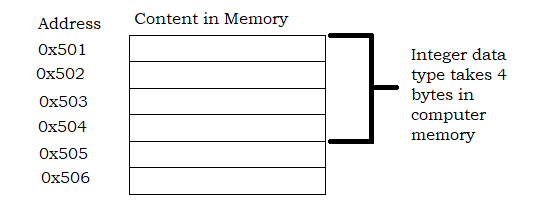A computerâ€™s memory is a collection of memory units (i.e. byte). When sizeof (int) returns four in a particular computer system, we can say that an integer variable takes 4 bytes to hold its value in that specific computer systemâ€™s memory. Also, please note that the return value of the sizeof operator also depends on the machines that you are using (32-bit system or 64-bit system).

### Syntax:

Sizeof(type)
Sizeof(expression)

The return type of sizeof is size_t.

### Examples:

Now since we understand the sizeof operator and know the syntax, let us look at a couple of examples, which will help us to understand the concept in a better way.

• Sizeof for built-in types (example1.c)
• Sizeof for Array (example2.c)
• Sizeof for user-defined types (example3.c)
• Sizeof for variables (example4.c)
• Sizeof for expression (example5.c)
• Practical usage of sizeof (example6.c)

### Sizeof for built-in types (example1.c):

In this program, we will see how the sizeof operator works for built-in data types such as int, char, float, double. Let us look at the program and output.

#include <stdio.h>

int main()
{
printf(“Size of char   =  %ld n, sizeof(char));
printf(“Size of int    =  %ld n, sizeof(int));
printf(“Size of float  =  %ld n, sizeof(float));
printf(“Size of double =  %ld nn, sizeof(double));

printf(“Size of short int      =  %ld n, sizeof(short int));
printf(“Size of long int       =  %ld n, sizeof(long int));
printf(“Size of long long int  =  %ld n, sizeof(long long int));
printf(“Size of long double    =  %ld n, sizeof(long double));

return 0;
}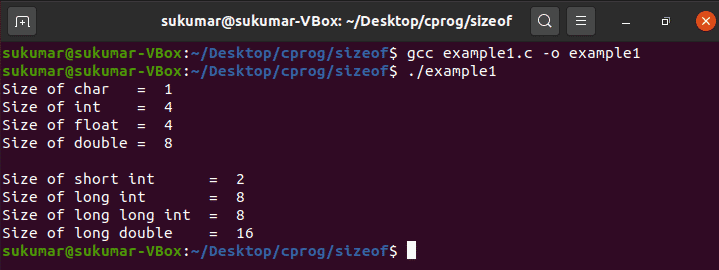### Sizeof for Array (example2.c)

In this program, we will see how to use the sizeof operator for different types of array. In case of an array, the sizeof operator will return (No. of elements in the array * Sizeof (array type)). For example, when we declare an integer type array of 10 elements (int SmartPhones  ;), the sizeof(Smartphones) will return:

(No. of elements in SmartPhones * sizeof(int)) = (10 * 4) = 40

Let us look at the program and output.

#include <stdio.h>

int main()
{
int SmartPhones;
char SmartPhoneNames;
double SmartPhonesPrice;

printf(“Size of int     =  %ld n, sizeof(int));
printf(“Size of char    =  %ld n, sizeof(char));
printf(“Size of double  =  %ld n, sizeof(double));

/* Find out the sizeof Array*/
printf(“Size of SmartPhones       =  %ld n, sizeof(SmartPhones));
printf(“Size of SmartPhoneNames   =  %ld n, sizeof(SmartPhoneNames));
printf(“Size of SmartPhonesPrice  =  %ld n, sizeof(SmartPhonesPrice));

return 0;
}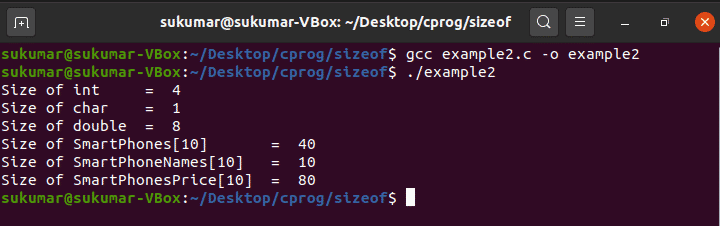### Sizeof for user-defined types(example3.c):

In this example, we will see how to use sizeof operator for user-defined data types such as structure and union. Letâ€™s use the program and understand the output.

Now, looking at the program, and we can manually calculate the size of SmartPhoneType. As you can see below, SmartPhoneType is a structure, and it contains the following elements:

• Number of character type variable = 1 [sp_name]
• Number of integer type variable= 1 [sp_version]
• Number of float type variables= 3 [sp_length, sp_width, sp_height]

From the example-1, we have seen that:

• Size of character is 1 byte
• Size of an integer is 4 bytes
• Size of a float is 4 bytes

Therefore, if we add up the size of all the elements in the structure, we should be able to get the size of the structure, i.e. SmartPhoneType. Therefore, the size of the structure should be = (1 + 4 + 4 + 4 + 4) bytes = 17 bytes. However, the program output says the structure size is 20. The extra 3 bytes (sp_name, which is a character, is taking 4 bytes instead of 1 byte) allocated for the structure due to the structure padding.

#include <stdio.h>

/* Create an user defined structure type – SmartPhoneType*/
struct SmartPhoneType
{
char  sp_name;
int   sp_version;
float sp_length;
float sp_width;
float sp_height;
}SmartPhone;

/* Define an user defined union type – SmartPhoneUnionType*/
Union SmartPhoneUnionType
{
char  sp_name;
int   sp_version;
float sp_length;
float sp_width;
float sp_height;
}SmartPhone_u;

int main()
{
/* Find out the size of structure and union*/
printf(“Size of struct   =  %ld n, sizeof(SmartPhone));
printf(“Size of union    =  %ld n, sizeof(SmartPhone_u));

return 0;
}### Sizeof for variables (example4.c):

This example program illustrates that the sizeof operator is capable of accepting the variable also and return the size of the variable.

#include <stdio.h>

int main()
{
/* Declare char, int, float and double type variable and array */
char   var_a, var_b;
int    var_c, var_d;
float  var_e, var_f;
double var_g, var_h;

/* Find out the size of variables and array.
This program demonstrates that variable can also
be used as an operand sizeof operator*/

/* size of char, char variable and char array*/
printf(“Size of char        =  %ld n, sizeof(char));
printf(“Size of var_a       =  %ld n, sizeof(var_a));
printf(“Size of var_b   =  %ld nn, sizeof(var_b));

/* size of int, int variable and int array*/
printf(“Size of int         =  %ld n, sizeof(int));
printf(“Size of var_c       =  %ld n, sizeof(var_c));
printf(“Size of var_d   =  %ld nn, sizeof(var_d));

/* size of float, float variable and float array*/
printf(“Size of float       =  %ld n, sizeof(float));
printf(“Size of var_e       =  %ld n, sizeof(var_e));
printf(“Size of var_f   =  %ld nn, sizeof(var_f));

/* size of double, double variable and double array*/
printf(“Size of double      =  %ld n, sizeof(double));
printf(“Size of var_g       =  %ld n, sizeof(var_g));
printf(“Size of var_h   =  %ld n, sizeof(var_h));

return 0;
}### Sizeof for expression(example5.c):

In this example program, we will demonstrate that the sizeof operator can also accept an expression and return the size of the resulting expression.

#include <stdio.h>

int main()
{
int    var_a = 5, var_b = 3;
double  var_c = 2.5, var_d = 4.5;

printf(“Size of int     =  %ld n, sizeof(int));
printf(“Size of double  =  %ld nn, sizeof(double));

printf(“Size of var_a * var_b   =  %ld n, sizeof(var_a * var_b));
printf(“Size of var_c * var_d   =  %ld n, sizeof(var_c * var_d));

/* Here we are multiplying an integer variable with a double variable.
Therefore, sizeof operator will return the size of maximum sized
variable i.e. double type variable.*/

printf(“Size of var_a * var_c   =  %ld n, sizeof(var_a * var_c));

return 0;
}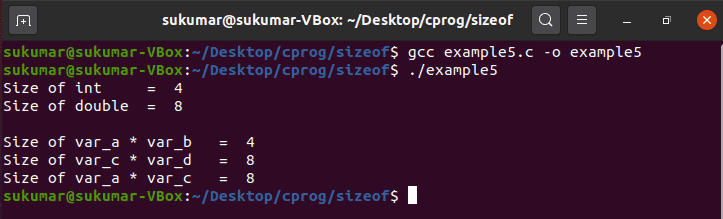### Practical usage of sizeof (example6.c):

This example program will help you to understand a practical use case of the sizeof operator. The Sizeof operator is very much useful while allocating the dynamic memory from heap using malloc. Let us look at the program and the output.

#include <stdio.h>
#include <stdlib.h>

typedef struct
{
char  sp_name;
int   sp_version;
float sp_length;
float sp_width;
float sp_height;
} SmartPhoneType;

int main()
{
/* Allocate memory in the Heap memory for holding five SmartPhoneType
variables.
*/

SmartPhoneType * SmartPhone_Ptr = (SmartPhoneType *)malloc(5 * sizeof(SmartPhoneType));

if(SmartPhone_Ptr != NULL)
{
printf(“Memory allocated for 5 SmartPhoneType structure variables in
the Heap memory.n
);

}
else
{
printf(“Error occured during the heap memory allocation!”);
}

return 0;
}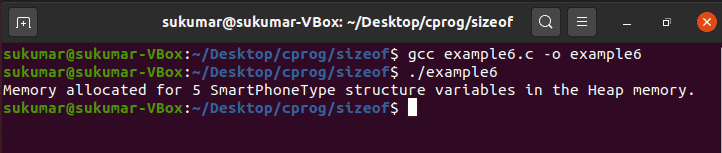### Conclusion:

The Sizeof is an important unary operator in the C programming language. It helps us in determining the size of primitive data types, user-defined data types, expressions, etc. in computer memory. The Sizeof operator plays an important role in allocating dynamic memory in C using malloc, calloc, etc. in the Heap memory.